June 06, 2023, 01:34:58 PM

## News:

IonicWind Snippit Manager 2.xx Released!  Install it on a memory stick and take it with you!  With or without IWBasic!

## Vector/Matrix library

Started by John S, January 01, 2007, 04:52:22 AM

0 Members and 1 Guest are viewing this topic.

#### John S

#####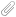January 01, 2007, 04:52:22 AMLast Edit: January 07, 2007, 12:00:17 PM by John S
Vectors are used in all sorts of programs from games to serious engineering applications.  Typically, column vectors are used.  Vectors are arrays of numbers (in this case double values) with the dimensions of n x 1 (n rows by 1 column).  The order of the values in the vector are important as each position in the vector represents a parameter in the vector space different than and orthogonal to ( perpendicular to ) the other positions.   Some examples:

A force acting in 3d space, a vector can be created such as:   F = ( Fx, Fy, Fz )        // shown as a row vector
An object flying across the computer screen (2d):   V = ( Vx, Vy )
A chemical reaction:  K = ( K1, K2, K3, K4, ... )

The only limitation associated with numbers of dimensions is related to the Cross Product method (CrossProd) which requires 3-d vectors at this time.

This include file contains several useful methods (functions) for dealing with vectors.  I will be using this as a foundation for a Vector and Matrix Math (Linear Algebra) library.  I may be adding additional methods related to plotting vectors and transform functions such as translating, rotating, transforming, scaling, ... as need arises.

So far I have the following functions/methods (these declares come straight from the include file):

declare DVector();             // class constructor
declare _DVector();            // class destructor

//member functions
declare virtual Get(int pos), double;          // get value at position
declare virtual Set(int pos, double value);    // set value at position

declare virtual Ins(int pos, int value);       // insert value at position
declare virtual ReSize(int size);              // resize vector
declare virtual GetCount(),int;                // get number of elements

declare ZeroAll();                             // reset all elements to zero
declare RemoveAll();                           // reset array to zero elements (none)

declare virtual Write(string title, string subtitle, string linetext, uint printflag);  // utility to help write out vectors

declare virtual ScalarProd(double value);      // scalar product A = sA

declare virtual Add(DVector B), uint;          // add vectors   A = A + B
declare virtual Subt(DVector B), uint;         // subtract vectors  A = A - B

declare virtual Copy(DVector B), uint;         // copy  A = B
declare virtual Copy2(DVector B), uint;        // copy  B = A
declare virtual Swap(DVector B), uint;         // swap  A <-> B

declare virtual Magnitude(), double;           // scalar magnitude of vector (a1^2 + a2^2 + a3^2 + ... )^0.5

declare virtual DotProd(DVector B), double;    // Dot Product of vectors (s = A(T) * B) result is scalar (double)

declare virtual AngleR(DVector B), double;     // Angle between two vectors is scalar (double) in radians
declare virtual AngleD(DVector B), double;     // Angle between two vectors is scalar (double) in degrees

declare virtual Add2(DVector B, DVector C), uint;   // add vectors   A = B + C
declare virtual Subt2(DVector B, DVector C), uint;  // subtract vectors A = B - C

declare virtual Distance(DVector B), double;   // scalar distance between two vectors ((a1-b1)^2 + (a2-b2)^2 + ... )^0.5

declare virtual NormalizeVector(DVector B), uint;   // generates the Normalized vector for B such that A = B/|B|   * revised to be consistant
declare virtual CrossProd(DVector B, DVector C), uint; // Cross Product of 3d vectors A = B x C results in column vector

declare ProjectOnVector(DVector B), uint;              // vector A = vector A projected onto Vector B
declare ProjectOnVector2(DVector B, DVector C), uint;  // vector A = vector B projected onto Vector C

declare virtual Orthogonal(DVector B), uint;           // vector A = Normalized vector orthogonal to B in direction of original A

declare virtual Orthogonal2(DVector B, DVector C), uint; // vector A = Normalized Vector orthogonal to B & C (3D only)
declare virtual Rotate3D(DVector B, double AX, double AY, double AZ), uint;  // vector A = vector B rotated about x, y & z axes (3D only - angles in radians)
declare virtual Rotate3DD(DVector B, double AX, double AY, double AZ), uint; // vector A = vector B rotated about x, y & z axes (3D only - angles in degrees)

//member variables
int m_mem;                    // pointer to vector's memory address
int m_GrowBy;                 // amount to grow memory by
int m_numItems;               // number of elements in the vector
int m_allocated;              // size of memory allocated

editted 01/06/07:   Cross (Vector) Product for vectors dimensioned only exist for 3D & 7D vector spaces.  Only the 3D vector space solution is included.  Due to time constraints, I will not code for the 7 dimensional Cross Product.  Anyone interested in Superstring Theory and needs that type of coding, I encourage you to do it and post it here.
John Siino, Advanced Engineering Services and Software

#### John S

#####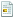January 06, 2007, 10:20:18 AM #1
I did some research and found that Cross (Vector) Products for vectors only exists with vecors dimensioned as 3 and 7 (2 also, but it points to 3 and/or translates to calculating the area of a parallelogram).   There are no other valid Cross Products in other dimensioned vector spaces.

Thinking about it brought to mind some interesting physical, mathematical and even philosophical concepts, but otherwise was not fruitful.  Due to time constraints, I will not code for the 7 dimensional Cross Product.  Anyone interested in Superstring Theory and needs that type of coding, I encourage you to do it and post it here.
John Siino, Advanced Engineering Services and Software

#### John S

#####January 06, 2007, 04:58:57 PM #2
Renamed UnitVector to NormalizeVector

declare virtual Copy2(DVector B), uint;                   // copy  B = A

declare ProjectOnVector(DVector B), uint;                     // vector A = vector A projected onto Vector B
declare ProjectOnVector2(DVector B, DVector C), uint;    // vector A = vector B projected onto Vector C

declare virtual Orthogonal(DVector B), uint;                    // vector A = unit vector orthogonal to B in direction of original A
John Siino, Advanced Engineering Services and Software

#### John S

#####January 07, 2007, 12:01:46 PM #3

declare virtual Orthogonal2(DVector B, DVector C), uint; // vector A = Normalized Vector orthogonal to B & C (3D only)
declare virtual Rotate3D(DVector B, double AX, double AY, double AZ), uint;  // vector A = vector B rotated about x, y & z axes (3D only - angles in radians)
declare virtual Rotate3DD(DVector B, double AX, double AY, double AZ), uint; // vector A = vector B rotated about x, y & z axes (3D only - angles in degrees)
John Siino, Advanced Engineering Services and Software

#### John S

#####March 29, 2007, 01:06:19 PM #4
There was a logic error in Paul's OOP.src file which I used as a foundation for my DVector source.  I am going to revise DVector and add more stuff hopefully this weekend.
John Siino, Advanced Engineering Services and Software

#### pistol350

#####March 30, 2007, 06:43:20 AM #5
hello John,
What do you mean by a "logic error"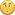I mean, as the code compiles well with no error, where is this error from?
Or maybe i don't understand what you mean.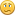Good job and thanks for sharing!

Cheers
_____________________________________________________________________________________________________
Peter
Regards,

Peter B.

#### Ionic Wind Support Team

#####March 30, 2007, 07:57:33 AM #6
Meaning my logic for allocating memory in blocks (growing) had a bug in it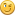So the problem wasn't a compile error.
Ionic Wind Support Team

#### John S

#####March 30, 2007, 01:45:48 PM #7
I'll upload revised files this weekend.  I got busy at work and school.
John Siino, Advanced Engineering Services and Software

#### pistol350

#####April 01, 2007, 02:59:12 PM #8
Thank you.Yeah,i understand better now!
Regards,

Peter B.

#### John S

#####April 11, 2007, 02:00:42 AM #9
Sorry for the delay, but here it is.  Please test this for me.  You may need to make slight modifications to use in projd\ect form.

I now am using dataVector as a base class for a vector of double values ( dataVector.src & dataVector.src ).   dVector now is a child class which inherits basic methods (resize, copy, etc.) and variables and adds vector math (linear algebra) methods.

If the user wants to change from double to float or int values, change the defined constant mlength to 4 in dataVector.src.

for double:
#define mlength 8          // mlength = 8 for double, 4 for int and float

for float or int:
#define mlength 4          // mlength = 8 for double, 4 for int and float

I would recommend renaming dataVector to idataVector or fdataVector.
John Siino, Advanced Engineering Services and Software